Home  /  Products  /  Stata 14  /  Bayesian analysis

# Bayesian analysis

## Highlights

• Bayesian estimation—thousands of built-in models, by combining
• 10 likelihood models
• 18 prior distributions
• Continuous, binary, ordinal, and count outcomes
• Univariate, multivariate, and multiple-equation models
• Linear models, nonlinear models, and generalized nonlinear models
• Continuous univariate, multivariate, and discrete priors
• Cannot find the model you need? Write your own.
• MCMC methods
• Full Gibbs sampling for some models
• Graphical tools to check MCMC convergence
• Posterior summaries: means, medians, SDs, MCSEs, CrIs
• Hypothesis testing: interval, model posterior probabilities
• Model comparison: DIC, Bayes factors
• Save your MCMC and estimation results for future use
See the complete list »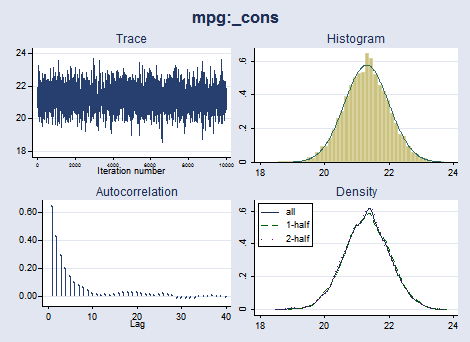×You may be interested in Bayesian analysis if

• you have some prior information available from previous studies that you would like to incorporate in your analysis. For example, in a study of preterm birthweights, it would be sensible to incorporate the prior information that the probability of a mean birthweight above 15 pounds is negligible.
• your research problem may require you to answer a question: What is the probability that my parameter of interest belongs to a specific range? For example, what is the probability that an odds ratio is between 0.2 and 0.5?
• you want to assign a probability to your research hypothesis. For example, what is the probability that a person accused of a crime is guilty?
• And more.

Stata 14 provides a new suite of features for performing Bayesian analysis. Stata's bayesmh fits a variety of Bayesian regression models using an adaptive Metropolis–Hastings (MH) Markov chain Monte Carlo (MCMC) method. Gibbs sampling is also supported for selected likelihood and prior combinations. Commands for checking convergence and efficiency of MCMC, for obtaining posterior summaries for parameters and functions of parameters, for hypothesis testing, and for model comparison are also provided.

## Let's see it work

Your Bayesian analysis can be as simple or as complicated as your research problem. Here's an overview.

## Normal model with known variance

Suppose we want to estimate the mean car mileage mpg. Our standard frequentist analysis may fit a regression model to mpg and look at the constant _cons.

. regress mpg

Source         SS           df       MS   Number of obs   =        74
F(0, 73)        =      0.00
Model            0         0           .   Prob > F        =         .
Residual   2443.45946        73  33.4720474   R-squared       =    0.0000
Total   2443.45946        73  33.4720474   Root MSE        =    5.7855

mpg        Coef.   Std. Err.      t    P>|t|     [95% Conf. Interval]

_cons     21.2973   .6725511    31.67   0.000      19.9569    22.63769



### Estimation

To fit a Bayesian model, in addition to specifying a distribution or a likelihood model for the outcome of interest, we must also specify prior distributions for all model parameters.

For simplicity, let's model mpg using a normal distribution with a known variance of, say, 35 and use a noninformative flat prior (with a density of 1) for the mean parameter {mpg:_cons}.

. bayesmh mpg, likelihood(normal(35)) prior({mpg:_cons}, flat)

Burn-in ...
Simulation ...

Model summary

Likelihood:
mpg ~ normal({mpg:_cons},35)

Prior:
{mpg:_cons} ~ 1 (flat)

Bayesian normal regression                       MCMC iterations  =     12,500
Random-walk Metropolis-Hastings sampling         Burn-in          =      2,500
MCMC sample size =     10,000
Number of obs    =         74
Acceptance  rate =      .4027
Log marginal likelihood = -233.90324             Efficiency       =      .1984

Equal-tailed
mpg       Mean   Std. Dev.     MCSE     Median  [95% Cred. Interval]

_cons   21.30713   .6933058   .015564   21.32559   19.91967   22.64948



bayesmh discarded the first 2,500 burn-in iterations and used the subsequent 10,000 MCMC iterations to produce the results. The estimated posterior mean, the mean of the posterior distribution, of parameter {mpg:_cons} is close to the OLS estimate obtained earlier, as is expected with the noninformative prior. The estimated posterior standard deviation is close to the standard error of the OLS estimate.

The MCSE of the posterior mean estimate is 0.016. The MCSE is about the accuracy of our simulation results. We would like it to be zero, but that would take an infinite number of MCMC iterations. We used 10,000 iterations and have results accurate to about 1 decimal place. That's good enough, but if we wanted more accuracy, we could increase the MCMC sample size.

According to the credible interval, the probability that the mean of mpg is between 19.92 and 22.65 is about 0.95. Although the confidence interval reported on our earlier regression has similar values, it does not have the same probabilistic interpretation.

Because bayesmh uses MCMC, a simulation-based method, the results will be different every time we run the command. (Inferential conclusions should stay the same provided MCMC converged.) You may want to specify a random-number seed for reproducibility in your analysis.

### Checking MCMC convergence

The interpretation of our results is valid only if MCMC converged. Let's explore convergence visually.

. bayesgraph diagnostics {mpg:_cons}, histopts(normal)The trace plot of {mpg:_cons} demonstrates good mixing. The autocorrelation dies off quickly. The posterior distribution of {mpg:_cons} resembles the normal distribution, as is expected for the specified likelihood and prior distributions. We have no reason to suspect nonconvergence.

We can now proceed with further analysis.

### Hypothesis testing

We can test an interval hypothesis that the mean mileage is greater than 21.

. bayestest interval {mpg:_cons}, lower(21)

Interval tests     MCMC sample size =    10,000

prob1 : {mpg:_cons} > 21

Mean    Std. Dev.      MCSE

prob1      .6735     0.46896   .0099939



The estimated probability of this interval hypothesis is 0.67. This is in contrast with the classical hypothesis testing that provides a deterministic decision of whether to reject the null hypothesis that the mean is greater than 21 based on some prespecified level of significance. Frequentist hypothesis testing does not assign probabilistic statements to the tested hypotheses.

### Informative priors

Suppose that based on previous studies, we have prior information that the mean mileage is normally distributed with mean 30 and variance 5. We can easily incorporate this prior information in our Bayesian model. We will also store our MCMC and estimation results for future comparison.

. bayesmh mpg, likelihood(normal(35)) prior({mpg:_cons}, normal(30,5))
saving(prior1_sim)

Burn-in ...
Simulation ...

Model summary

Likelihood:
mpg ~ normal({mpg:_cons},35)

Prior:
{mpg:_cons} ~ normal(30,5)

Bayesian normal regression                       MCMC iterations  =     12,500
Random-walk Metropolis-Hastings sampling         Burn-in          =      2,500
MCMC sample size =     10,000
Number of obs    =         74
Acceptance  rate =       .409
Log marginal likelihood = -242.58274             Efficiency       =       .209

Equal-tailed
mpg       Mean   Std. Dev.     MCSE     Median  [95% Cred. Interval]

_cons    22.0617   .6683529   .014619   22.05628   20.75121   23.39481

file prior1_sim.dta saved

. estimates store prior1


This prior resulted in a slight increase of the posterior mean estimate—the prior shifted the estimate toward the specified prior mean of 30.

Suppose that another competing prior is that the mean mileage is normally distributed with mean 20 and variance 4.

. bayesmh mpg, likelihood(normal(35)) prior({mpg:_cons}, normal(20,4))
saving(prior2_sim)

Burn-in ...
Simulation ...

Model summary

Likelihood:
mpg ~ normal({mpg:_cons},35)

Prior:
{mpg:_cons} ~ normal(20,4)

Bayesian normal regression                       MCMC iterations  =     12,500
Random-walk Metropolis-Hastings sampling         Burn-in          =      2,500
MCMC sample size =     10,000
Number of obs    =         74
Acceptance  rate =      .4113
Log marginal likelihood =  -235.7438             Efficiency       =      .2043

Equal-tailed
mpg       Mean   Std. Dev.     MCSE     Median  [95% Cred. Interval]

_cons   21.17991   .6658923   .014733   21.17731   19.87617   22.47459

file prior2_sim.dta saved

. estimates store prior2


The results using this prior are more similar to the earlier results with the noninformative prior.

### Model comparison

We can compare our two models that used different informative priors. Estimation results of the models were stored under prior1 and prior2. To compare the models, we type

. bayesstats ic prior1 prior2

Bayesian information criteria

DIC    log(ML)    log(BF)

prior1   472.0359  -242.5827          .
prior2   470.8157  -235.7438   6.838942

Note: Marginal likelihood (ML) is computed
using Laplace-Metropolis approximation.


The second model has a lower DIC value and is thus preferable.

Bayes factors—log(BF)—are discussed in [BAYES] bayesstats ic. All we will say here is that the value of 6.84 provides very strong evidence in favor of our second model, prior2.

We can also compute posterior probabilities for each model.

. bayestest model prior1 prior2

Bayesian model tests

log(ML)       P(M)     P(M|y)

prior1  -242.5827     0.5000     0.0011
prior2  -235.7438     0.5000     0.9989

Note: Marginal likelihood (ML) is computed using
Laplace-Metropolis approximation.


The posterior probability of the first model is very low compared with that of the second model. In fact, the posterior probability of the first model is near 0, whereas the posterior probability of the second model is near 1.

## Normal model with unknown variance

Continuing our car-mileage example, we now relax the assumption of a known variance of the normal distribution and model it as a parameter {var}. We specify a noninformative Jeffreys prior for the variance parameter.

. bayesmh mpg, likelihood(normal({var})) prior({mpg:_cons}, flat)
prior({var}, jeffreys)

Burn-in ...
Simulation ...

Model summary

Likelihood:
mpg ~ normal({mpg:_cons},{var})

Priors:
{mpg:_cons} ~ 1 (flat)
{var} ~ jeffreys

Bayesian normal regression                       MCMC iterations  =     12,500
Random-walk Metropolis-Hastings sampling         Burn-in          =      2,500
MCMC sample size =     10,000
Number of obs    =         74
Acceptance  rate =      .2883
Efficiency:  min =     .08843
avg =      .1191
Log marginal likelihood = -234.63956                          max =      .1499

Equal-tailed
Mean   Std. Dev.     MCSE     Median  [95% Cred. Interval]

mpg
_cons   21.30678   .7018585   .023602   21.30086   19.91435   22.72222

var   34.38441   5.787753   .149506   33.73722   24.71946    47.7112



Note that the MCSE for parameter {mpg:_cons} is larger in this model than it was in the model with a fixed variance. As the number of model parameters increases, the efficiency of the MH algorithm decreases, and the task of constructing an efficient algorithm becomes more and more important. In the above, for example, we could have improved the efficiency of MH by specifying the variance parameter in a separate block, block({var}), to be sampled independently of the mean parameter.

Even without adding the blocking, convergence diagnostics for both mean and variance look good.

. bayesgraph diagnostics _all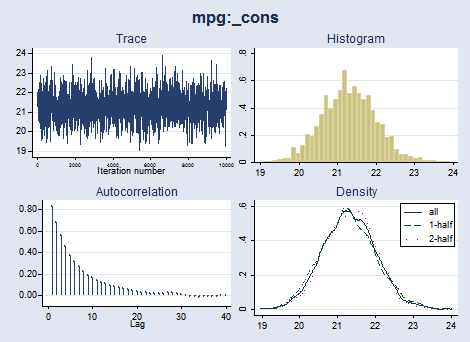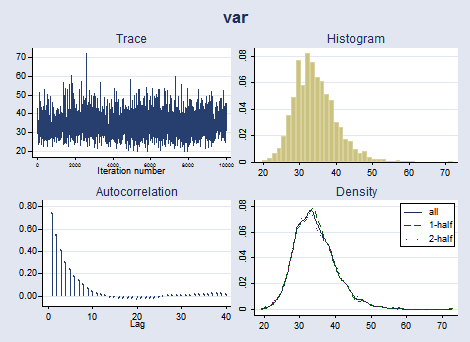We can compute summaries for linear and nonlinear expressions of our parameters. Let's compute summaries for a standardized mean, which is a function of both the mean parameter and the variance parameter.

. bayesstats summary (mean_std: {mpg:_cons}/sqrt({var}))

Posterior summary statistics                      MCMC sample size =    10,000

mean_std : {mpg:_cons}/sqrt({var})

Equal-tailed
Mean   Std. Dev.     MCSE     Median  [95% Cred. Interval]

mean_std   3.670299   .3183261   .008546   3.661119   3.060899   4.308195



## Simple linear regression

bayesmh makes it easy to include explanatory variables in our Bayesian models. The syntax for regressions looks just as it does in other Stata estimation commands. For example, we can include an indicator of whether the car is foreign or domestic when modeling the mean car mileage.

. bayesmh mpg foreign, likelihood(normal({var}))
prior({mpg:_cons foreign}, flat)
prior({var}, jeffreys)

Burn-in ...
Simulation ...

Model summary

Likelihood:
mpg ~ normal(xb_mpg,{var})

Priors:
{mpg:foreign _cons} ~ 1 (flat)                                           (1)
{var} ~ jeffreys

(1) Parameters are an element of the linear form xb_mpg.

Bayesian normal regression                       MCMC iterations  =     12,500
Random-walk Metropolis-Hastings sampling         Burn-in          =      2,500
MCMC sample size =     10,000
Number of obs    =         74
Acceptance  rate =       .188
Efficiency:  min =     .06344
avg =     .06893
Log marginal likelihood = -227.16451                          max =     .07414

Equal-tailed
Mean   Std. Dev.     MCSE     Median  [95% Cred. Interval]

mpg
foreign   4.987455    1.43297   .054471   5.014443   2.034135   7.775843
_cons   19.81477   .7796195   .030952   19.79542   18.27116   21.35802

var   29.52163   5.304377   .194809   28.82301   20.82704   41.50129



We specified a flat prior for both the constant and the coefficient of foreign.

## Multivariate linear regression

We can fit a multivariate normal regression to model two size characteristics of automobiles, —trunk space, trunk, and turn circle, turn,— as a function of where the car is manufactured, foreign, foreign or domestic. The syntax for the regression part of the model is just like the syntax for Stata's mvreg (multivariate regression) command.

We model the covariance matrix of trunk and turn as the matrix parameter {Sigma,matrix}. We specify noninformative normal priors with large variances for all regression coefficients and use Jeffreys prior for the covariance. The MH algorithm has very low efficiencies for sampling covariance matrices, so we use Gibbs sampling instead. The regression coefficients are sampled by using the MH method.

. bayesmh trunk turn = foreign, likelihood(mvnormal({Sigma, matrix}))
prior({trunk:} {turn:}, normal(0,1000))
prior({Sigma, matrix}, jeffreys(2))
block({Sigma, matrix}, gibbs)

Burn-in ...
Simulation ...

Model summary

Likelihood:
trunk turn ~ mvnormal(2,xb_trunk,xb_turn,{Sigma,m})

Priors:
{trunk:foreign _cons} ~ normal(0,1000)                                   (1)
{turn:foreign _cons} ~ normal(0,1000)                                   (2)
{Sigma,m} ~ jeffreys(2)

(1) Parameters are an element of the linear form xb_trunk.
(2) Parameters are an element of the linear form xb_turn.

Bayesian multivariate normal regression          MCMC iterations  =     12,500
Metropolis-Hastings and Gibbs sampling           Burn-in          =      2,500
MCMC sample size =     10,000
Number of obs    =         74
Acceptance  rate =      .5998
Efficiency:  min =     .05162
avg =      .3457
Log marginal likelihood =  -410.2743                          max =      .7758

Equal-tailed
Mean   Std. Dev.     MCSE     Median  [95% Cred. Interval]

trunk
foreign  -3.348432   1.056294   .046491  -3.337957  -5.417821  -1.375893
_cons   14.75301   .5450302   .019877   14.73202   13.73661   15.89116

turn
foreign  -6.004471   .8822641   .038093  -5.991697  -7.751608  -4.267331
_cons   41.42375   .4817673   .017979   41.42091   40.48585   42.42505

Sigma_1_1   17.11048   3.028132   .036471   16.77325   12.21387   23.93075
Sigma_2_1   7.583515   2.026102   .024179    7.39855   4.153189   12.07798
Sigma_2_2     12.537   2.175963   .024705   12.29787   9.014795   17.45602



## Nonlinear model: Change-point analysis

As an example of a nonlinear model, we consider a change-point analysis of the British coal-mining disaster dataset for the period of 1851 to 1962. This example is adapted from Carlin, Gelfand, and Smith (1992). In these data, the count variable records the number of disasters involving 10 or more deaths.

The graph below suggests a fairly abrupt decrease in the rate of disasters around the 1887–1895 period.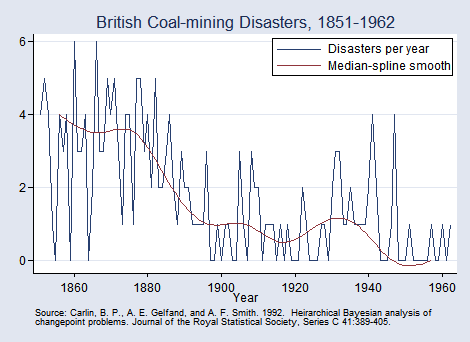Let's estimate the date when the rate of disasters changed.

We will fit the model

count ~ Poisson(mu1), if year < cp
count ~ Poisson(mu2), if year >= cp

cp—the change point—is the main parameter of interest.

We will use noninformative priors for the parameters: flat priors for the means and a uniform on [1851,1962] for the change point.

We will model the mean of the Poisson distribution as a mixture of mu1 and mu2.

. bayesmh count, likelihood(dpoisson({mu1}*sign(year<{cp})+{mu2}*sign(year>={cp})))
prior({mu1 mu2}, flat)
prior({cp}, uniform(1851,1962))
initial({mu1 mu2} 1 {cp} 1906)

Burn-in ...
Simulation ...

Model summary

Likelihood:
count ~ poisson({mu1}*sign(year<{cp})+{mu2}*sign(year>={cp}))

Hyperpriors:
{mu1 mu2} ~ 1 (flat)
{cp} ~ uniform(1851,1962)

Bayesian Poisson model                           MCMC iterations  =     12,500
Random-walk Metropolis-Hastings sampling         Burn-in          =      2,500
MCMC sample size =     10,000
Number of obs    =        112
Acceptance  rate =      .2281
Efficiency:  min =     .03747
avg =     .06763
Log marginal likelihood = -173.29271                          max =      .1193

Equal-tailed
Mean   Std. Dev.     MCSE     Median  [95% Cred. Interval]

mu1   3.118753   .3001234   .015504   3.110907   2.545246    3.72073
cp   1890.362     2.4808   .071835   1890.553   1886.065   1896.365
mu2   .9550596   .1209208   .005628   .9560248   .7311639   1.219045



The change point is estimated to have occurred in 1890 with the corresponding 95% CrI of [1886,1896].

We may also be interested in estimating the ratio between the two means.

. bayesstats summary (ratio: {mu1}/{mu2})

Posterior summary statistics                      MCMC sample size =    10,000

ratio : {mu1}/{mu2}

Equal-tailed
Mean   Std. Dev.     MCSE     Median  [95% Cred. Interval]

ratio   3.316399   .5179103   .027848   3.270496   2.404047   4.414975



After 1890, the mean number of disasters decreased by a factor of about 3.3 with a 95% credible range of [2.4, 4.4].

The interpretation of our change-point results is valid only if MCMC converged. We can explore convergence visually.

. bayesgraph diagnostics {cp} (ratio: {mu1}/{mu2})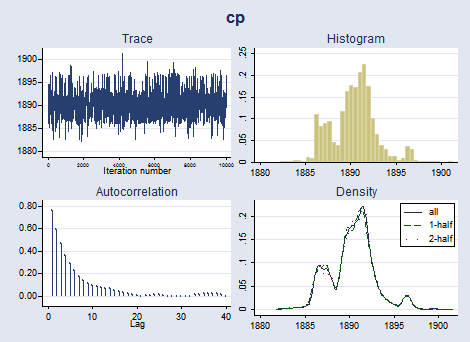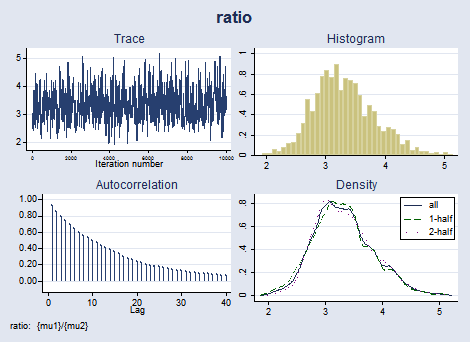The graphical diagnostics for {cp} and the ratio look reasonable. The marginal posterior distribution of the change point has the main peak at about 1890 and two smaller bumps around the years 1886 and 1896, which correspond to local peaks in the number of disasters.

## Using the GUI to perform Bayesian analysis

In Multivariate linear regression, we showed you how to use the command line to fit a Bayesian multivariate regression. Watch Graphical user interface for Bayesian analysis to see how to fit this model and more using the GUI.

## Reference

Carlin, B. P., A. E. Gelfand, and A. F. M. Smith. 1992. Hierarchical Bayesian analysis of changepoint problems. Journal of the Royal Statistical Society, Series C 41: 389–405.

## Tell me more

Stata's new Bayesian analysis features are documented in their own new 255-page manual. You can read more about Bayesian analysis, more about Stata's new Bayesian features, and see many worked examples in Stata Bayesian Analysis Reference Manual.

Read the Stata Blog entries Bayesian modeling: Beyond Stata's built-in models and Gelman–Rubin convergence diagnostic using multiple chains.